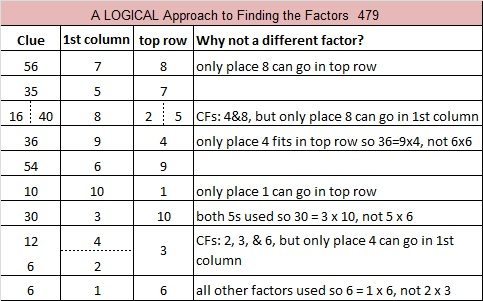479 and Level 6

479 is the sum of nine consecutive prime numbers.

37 + 41 + 43 + 47 + 53 + 59 + 61 + 67 + 71 = 479.Print the puzzles or type the solution on this excel file: 10 Factors 2015-04-27

—————————————————————————————————

• 479 is a prime number.
• Prime factorization: 479 is prime.
• The exponent of prime number 479 is 1. Adding 1 to that exponent we get (1 + 1) = 2. Therefore 479 has exactly 2 factors.
• Factors of 479: 1, 479
• Factor pairs: 479 = 1 x 479
• 479 has no square factors that allow its square root to be simplified. √479 ≈ 21.88607How do we know that 479 is a prime number? If 479 were not a prime number, then it would be divisible by at least one prime number less than or equal to √479 ≈ 21.8861. Since 479 cannot be divided evenly by 2, 3, 5, 7, 11, 13, 17, or 19, we know that 479 is a prime number.

—————————————————————————————————